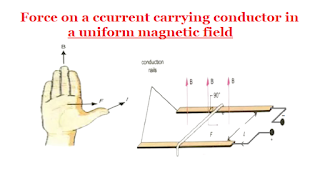Question: Define magnetic force and uniform magnetic field. Derive expression for the force acting on a current carrying conductor in a uniform magnetic field. Define B and its unit tesla ?Force on a ccurrent carrying conductor in a uniform magnetic field

MAGNETIC FORCE

The force exerted by magnet on another magnet.or magnetic material is called magnetic force. It is denoted by F

UNIFORM MAGNETIC FIELD

When magnetic Lines of force are parallel and uniformly spaced. Such a field is called uniform mgnetic field.

EXPRESSION FOR FORCE ON THE CONDUCTOR

When a current carrying conductor is placed in an external magnetic field, the magnetic field of the conductor interacts with the external field and conductor experiences a force. Consider a copper-rod of length 'L' when current 'I' is passed through it from the battery, the rod moves on the copper rails. The magnetic field B is vertically upward. The magnetic force on the conductor is perpendicular to the conductor and magnetic field B. All are shown in the figure.

The magnitude of the force depends upon the following factors.

(i) F ∝ Sin ∝ → 1

The force F is directly proportional to Sin ∝ where ∝  a is the angle between the conductor and field.

This shows the force is zero when the rod is parallel to the field and is maximum when conductor is placed prependicular to the field.

(ii) F ∝ I → 2

The force is directly proportional to the current flowing through the conductor. The more the current greater is the force.

(iii) F ∝ → 3

The force is directly proportional to the length of the conductor inside the magnetic field.

(iv) F ∝  B → 4

The force is directly proportional to the strength of the applied magnetic field B. The stronger the field, greater is the force, on combining all these factors, we get

F ∝  ILB Sin ∝

⇒ F = KILB Sin ∝

Where K is the constant of proportionality. In SI units its value is 1. Then

F = KILB Sin ∝ → 5

This equ. 5 gives the magnitude of force on current carrying conductor.

In vector form:

F = I ( L ✖️ B ) →  6

Where L = vector length of the conductor in the direction of current flow. The direction of the force F is given by the right hand rule of the cross-produet of vectors I and B. Rotate I towards through the smaller angle. Curl the fingers of right hand in the direction of rotation. The thumb points in the direction of force.

STRENGTH OF MAGNETIC FIELD (B)

From equ. 5:

F = KILB Sin ∝

⇒ B = F / IL Sin ∝

The force acting on per unit length of the conductor placed at right angle to the magnetic field when one ampere current passes through it.

Unit:

B = F / IL = 1N / 1A ✖️ 1m

B = 1 N A-1 m-1 = 1 Tesla

TESLA

When a force of  one Newton is exterted on one meter length of the conductor placed prependicular to the field and one ampere current passes through it.

CONVENTION TO REPRESENT DIRECTION

(i) OUT OF PAGE

The direction of current flowing out of the page (towards the reader) is represented by a symbol (•).

(ii) IN TO PAGE

The direction of current flowing into the page, (away from reader) is represented by a symbol (x).

DIRECTION OF FORCE ON A CONDUCTOR IN THE MAGNETIC FIELD

Consider the lines of magnetic force in the figure. The two fields tends to reinforce each other on the conductor and cancel each other on the right side of it. The conductor tends to move towards the weaker part of the field. The force on the conductor will be directed towards right prependicular to both the conductor and the magnetic field.